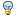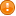(Do in on sugon server)

• do not forget to use compile script

• …or static linking: cc -O0 -static -mno-abicalls -mno-gpopt your_file.c -o your_program_name

• printf("%p\n", &variable) — print pointer to variable, which in C strictly means address

• write a program that prints addresses of a pair of local and a pair of global variables
• compare the addresses by sight
•interpret this

•unlike .data segment in MARS, real world .rdata segment is not at 0x1001000, but just after .text segment ends!)

• int *P; — «pointer to integer» variable type (i. e. address of memory part that keeps an integer value)

• Array name is array address, it equals to the array zero element address
• i. e. A == &A

• Address arithmetic. Write a program, that
• prints character (char) array zero and fifths element addresses

• prints integer (int) array zero and fifths element addresses

•interpret this

• Compare two global arrays A and B addresses by just subtracting them!

• ```   1 #include <stdio.h>
2
3 int A={1,2,3,4,5};
4 int B={9,8,7,6,5};
5
6 int main(int argc, char *argv[]) {
7   printf("A, B, B-A: %p, %p, %d\n", A, B, B-A);
8   return 0;
9 }
```
•Why B-A = 5?

• How to calculate real distance between A and B? Try it.

1.with sizeof() ?

2.with (int) cast ?

•Unexpected: remove both «={…}» initializers and compile code dynamically (cc prog.c -o prog)

• There's a chance that A address became larger than B! This is because the order of uninitialized elements in memory is not guaranteed

• Alignment. Compile this:
```   1 #include <stdio.h>
2 struct STRU {
3   int a;
4   int b;
5   double f;
6 };
7
8 struct STRU Massive;
9 void main(void) {
10   int i=3, j=4;
11   printf("Size of STRU: %d, STRU: %d\n", sizeof(struct STRU), sizeof(Massive));
12   printf("%d, %lf\n", Massive[i].a, Massive[j].f);
13   printf("%p, %p, %p\n", &Massive[i].a, &Massive[i].b, &Massive[i].f);
14
15 }
```
• Rewrite the structure as this:
```   1 struct STRU {
2   int a;
3   double f;
4   int b;
5 };
```
• compile and run
•interptet changes!

• (this is because alignment, see .align instructions in assrmber code)

HSE/ProgrammingOS/Lab_05_Addressing (last edited 2020-02-11 19:51:12 by FrBrGeorge)

• Page.execute = 0.022s
• getACL = 0.020s
• init = 0.001s